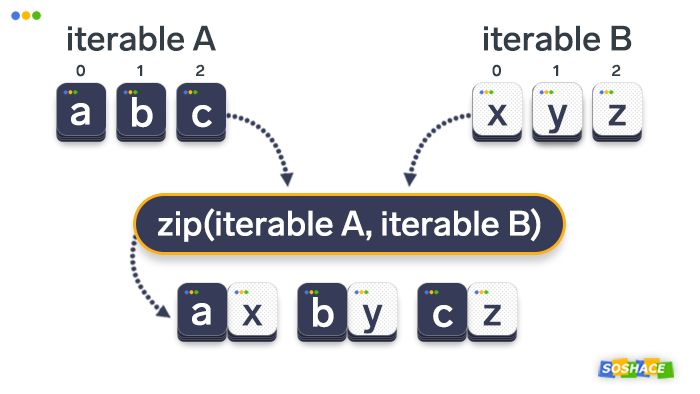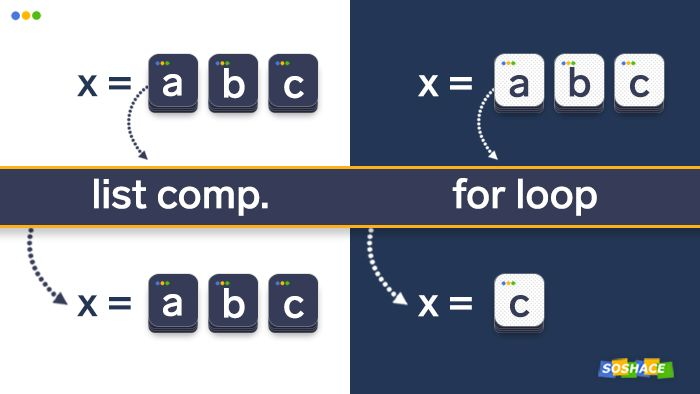# Python中zip()函数的解释和可视化

2019/04/10 10:10## zip()的作用

``````zip(iter1 [,iter2 [...]]) —> zip object
``````

Python的内置help()模块提供了一个简短但又有些令人困惑的解释：

``````uppercase = ['A', 'B', 'C']
lowercase = ['a', 'b', 'c']

for x, y in zip(uppercase, lowercase):
print(x, y)
``````

``````A a
B b
C c
``````

``````uppercase = ['A', 'B', 'C']
lowercase = ['a', 'b', 'c']
numbers = [1, 2, 3]

for x, y, z in zip(uppercase, lowercase, numbers):
print(x, y, z)
``````

``````A a 1
B b 2
C c 3
``````zip()函数的另一个重要警告是如果各个迭代器的元素个数不一致，则返回列表长度与最短的对象相同：

``````uppercase = ['A', 'B', 'C', 'D', 'E']
lowercase = ['a', 'b', 'c', 'd']
numbers = [1, 2, 3]

for x, y, z in zip(uppercase, lowercase, numbers):
print(x, y, z)
``````

``````A a 1
B b 2
C c 3
``````

• Iteration是计算机科学的通用术语。它是指对一组元素执行一项操作，一次执行一个元素。一个很好的例子是循环 -它适用于每个单独的项目，直到整个项目集运行完毕为止。
• Iterable是可以遍历的对象（译者注：在Python中所有东西都是object, 比如说变量，容器，类），iterable是可以产生iterator的object。
• iterator是表示数据流的对象，它一次返回一个元素的数据。它还会记住其在迭代过程中的位置。本质上，它控制应如何迭代可迭代对象

## 将zip()对象转换为列表（并使用索引）

zip()函数返回一个zip对象（类似于map()操作方式）。

zip对象提供了一些有趣的功能（迭代速度比list更快），但是我们经常需要将其转换为list。为了做到这一点，我们需要调用list()函数：

``````b = ["red", "green", "blue"]
c = ["leopard", "cheetah", "jaguar"]

print(list(zip(b, c)))
``````

``````[('red', 'leopard'), ('green', 'cheetah'), ('blue', 'jaguar')]
``````

list()函数将zip对象转换为一个元组列表。我们可以使用索引浏览各个元组。出于可读性考虑，我们首先将新列表分配给一个变量：

``````b = ["red", "green", "blue"]
c = ["leopard", "cheetah", "jaguar"]
new_list = list(zip(b, c))
print(new_list)
print(new_list)
print(new_list)
``````

``````('red', 'leopard')
('green', 'cheetah')
('blue', 'jaguar')
``````

## 将zip（）对象转换成字典

``````b = ["red", "green", "blue"]
f = ["strawberry", "kiwi", "blueberry"]

print(dict(zip(b, f)))
``````

``````{'red': 'strawberry', 'green': 'kiwi', 'blue': 'blueberry'}
``````

## 解压列表

``````a = [1, 2, 3]
b = [4, 5, 6]
zipped = zip(a, b)
list(zipped)

a2, b2 = zip(*zip(a, b))
print(a == list(a2) and b == list(b2))
``````

``````True
``````

## Zip与列表生成式（for循环潜在问题）

zip（）函数与Python中的for循环一起使用的可视化Python的另一个很棒的功能——列表推导式，可以与zip()函数结合使用。表面上看起来很简单……

``````m = ["mind", "mouse", "mini"]
n = ["norm", "night", "necklace"]

[print(a, b) for a, b in zip(m, n)]
``````

``````mind norm
mouse night
mini necklace
``````

``````Traceback (most recent call last):
File "C:\Pro\Py\tp-ex\tmp1.py", line 5, in
print(a)
NameError: name 'a' is not defined
``````

``````m = ["mind", "mouse", "mini"]
n = ["norm", "night", "necklace"]

for m, n in zip(m, n):
print(m, n)

print(m)
``````

``````mind norm
mouse night
mini necklace
mini
``````

## 结论

0
0 收藏

### 作者的其它热门文章0 评论
0 收藏
0